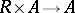# Topological algebra

(diff) ← Older revision | Latest revision (diff) | Newer revision → (diff)

A universal algebra that is a topological space such that the algebraic operations are continuous.

An algebra (in the sense of a "ring with operators" )over a topological field or commutative ringthat is a topological space in which the operations of addition and multiplication, as well as the mapping(), are continuous. A Banach algebra is an example of a topological algebra over the field of complex numbers.

The branch of algebra which studies topological algebraic structures, i.e. groups, semi-groups, rings, lattices, vector spaces, modules, and others, equipped with topologies in which the algebraic operations considered are continuous.

The notion of a topological group arose in connection with the study of groups of continuous transformations. Thus, in the second half of the 19th century, S. Lie and his school developed the theory of an important class of topological groups (groups of differentiable transformations from a manifold into itself), which were subsequently called Lie groups (cf. Lie group). The study of general topological groups began in the 1920's (cf. , ). By the early 1930's, topological groups, rings and fields were being systematically investigated.

A.N. Kolmogorov  developed an axiomatic approach to the study of topological projective geometries. Their classification depends essentially on the description of locally compact skew-fields (cf. Locally compact skew-field). A complete description of connected locally compact skew-fields was given in 1932 by L.S. Pontryagin (cf. , Chapt. 4).

Numerous problems in analysis led to the general definition of a Banach space (cf. also ), which motivated the systematic study of topological modules over topological rings and Banach algebras (cf. , ).

The basic branches of topological algebra at present are: topological groups and their generalizations (in particular, topological semi-groups and quasi-groups, cf. Topological group); topological rings (cf. Topological ring) (in particular, topological fields and skew-fields) and topological modules (cf. Topological module) over them (in particular, topological vector spaces, cf. Topological vector space); topological lattices (in particular, topological projective planes); and topological universal algebras (cf. , , , ).

The following lines of research in topological algebra can be distinguished: the existence of topologies in algebraic systems (groups, rings and others), to transform them into topological algebraic systems with special properties; questions about extending topologies to extensions of algebraic systems, and the possibility of imbedding them into topological algebraic systems of specific classes; properties of the topology of a topological algebraic system, in particular the possibility of specifying the topology by a metric or norm; the construction of different classes of topological algebraic systems (including the theory of radicals of a topological algebraic system); free topological algebraic systems; and questions of duality of topological algebraic systems.

How to Cite This Entry:
Topological algebra. Encyclopedia of Mathematics. URL: http://encyclopediaofmath.org/index.php?title=Topological_algebra&oldid=14332
This article was adapted from an original article by V.I. ArnautovA.V. Mikhalev (originator), which appeared in Encyclopedia of Mathematics - ISBN 1402006098. See original article